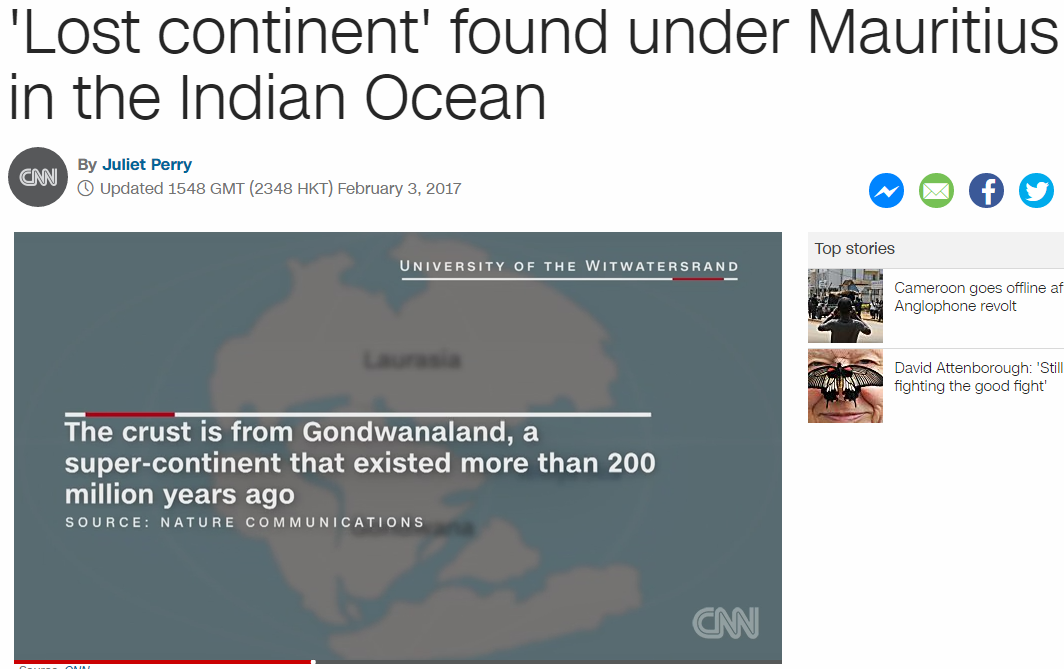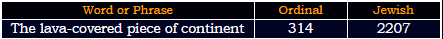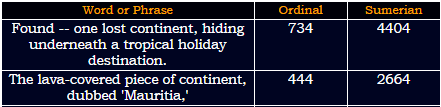CipherFeb 4, 2017

CNN - 'Lost continent' found under Mauritius in the Indian Ocean

CNN's story - http://edition.cnn.com/2017/02/03/world/lost-continent-mauritius-trnd/index.html'Lost continent' found under Mauritius in the Indian Ocean = 3+6+1+2+3+6+5+2+9+5+5+5+2+6+6+3+5+4+3+5+4+5+9+4+1+3+9+9+2+9+3+1+9+5+2+8+5+9+5+4+9+1+5+6+3+5+1+5 = 227 (Reduced)
Twenty-two divided by seven = 20+23+5+14+20+25+20+23+15 + 4+9+22+9+4+5+4 + 2+25 + 19+5+22+5+14 = 314 (Ordinal)
In the past few days there has been incredible amount of Pi and inverse Pi coding and they start this post by 227, later in the post there's 314 appearing as well

There's 1 related story that they highlight for usThe story was released on 1/28/2013, a date that corresponds to 2/3/2017, the release date of this story
2/3/2017 - 2 + 3 + 20 + 17 = 42
1/28/13 - 1 + 28 + 13 = 42
Also the only name appearing in the story coming today is Lewis Ashwal
Lewis Ashwal = 3+5+5+9+1 + 1+1+8+5+1+3 = 42 (Reduced)

But there's another connection between these 2 dates47 is one of the special numbers that is coded almost everywhere and it has some relation to this date and story (Foundation = 47, Framework = 47, Star of David = 47)

In this story they talk about Gondwanaland (continent that existed 200 million years ago) and how this "lost contient" is made from it's crust
Gondwanaland = 7+6+5+4+5+1+5+1+3+1+5+4 = 47 (Reduced)
2/3/2017 = 2 + 3 + 2 + 0 + 1 + 7 = 15 (47 is 15th prime number)
Four years = 6+6+3+9 + 7+5+1+9+1 = 47 (Reduced)
February Third = 6+5+2+9+3+1+9+7 + 2+8+9+9+4 = 74 (Reduced)

Here is the first part of the storyContinent contains rocks that are 3.6 billion years old = 223 (S Exception)
Mineral zircon in beach sand provided clue to continent 232 (Reduced)

The Synagogue of Satan = 20+8+5 + 19+25+14+1+7+15+7+21+5 + 15+6 + 19+1+20+1+14 = 223 (Ordinal)
Satan = 90+1+100+1+40 = 232 (Jewish)
They mention 3.6 billion years - 36th triangular number is 666 and there's 1 more 666 that will appear in the end of their story
Also we are only missing 322 to complete 777 (223+232+322 = 777)

They open the story with this
Found -- one lost continent, hiding underneath a tropical holiday destination. = 311 (Reduced)
Whenever you see this sort of weird writing usually there's something to be found
64th prime number is 311 (Israel = 64, Zion = 64, Thelema = 64, Leaders =64)

super-continent of Gondwana = 1+3+7+5+9+3+6+5+2+9+5+5+5+2 + 6+6 + 7+6+5+4+5+1+5+1 = 113 (Reduced) - supposedly existed 200 million years ago
two hundred million years = 2+5+6 + 8+3+5+4+9+5+4 + 4+9+3+3+9+6+5 + 7+5+1+9+1 = 113 (Reduced)

The lava-covered piece of continent, dubbed 'Mauritia,' was found under the popular island of Mauritius.It's very rare to see 314 and 227 in the same phraselava = 20+1+700+1 = 722 (Jewish) - the title of their post is 227, talking about a 'lava-covered piece of contient'

The team made the discovery by analyzing a mineral -- zircon -- found in rocks spewed up by lava during volcanic eruptions.
lava = 72+6+132+6 = 216 (Sumerian)
volcanic eruptions = 22+15+12+3+1+14+9+3 + 5+18+21+16+20+9+15+14+19 = 216 (Ordinal)

lava = 12+1+22+1 = 36 (Ordinal) - rocks they found are supposedly 3.6 billion years old

This is the rest of the storyThey finish with 666 for good measure